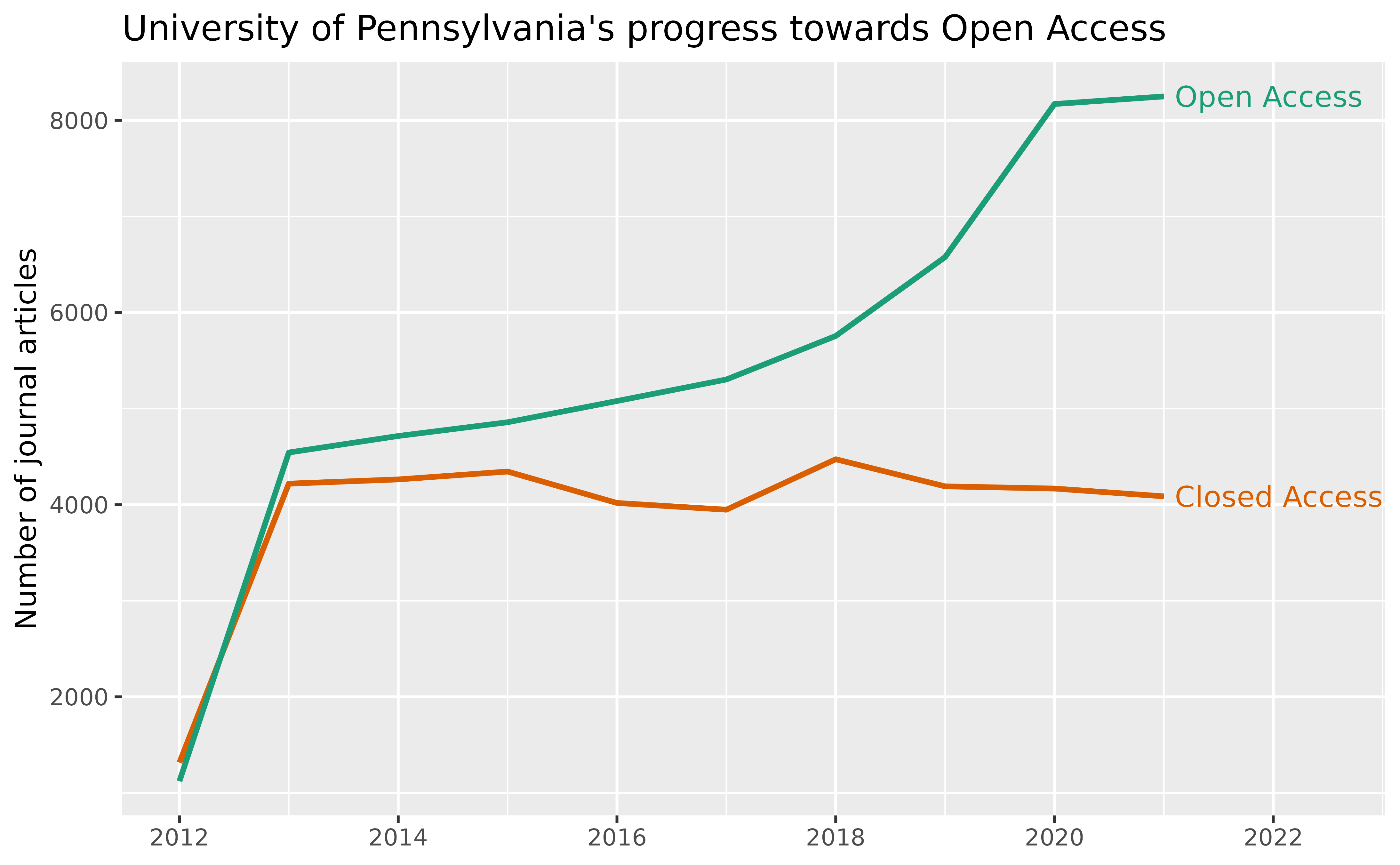Following the template in OpenAlex’s oa-percentage tutorial, this vignette uses openalexR to answer:

How many of recent journal articles from the University of Pennsylvania are open access? And how many aren’t?

``````library(openalexR)
library(dplyr)
library(tidyr)
library(ggplot2)``````

We first need to find the openalex.id for University of Pennsylvania. We can do this by fetching for the institutions `entity` and put “University of Pennsylvania” in `display_name` or `display_name.search`:

``````oa_fetch(
entity = "inst", # same as "institutions"
display_name.search = "\"University of Pennsylvania\""
) %>%
select(display_name, ror) %>%
knitr::kable()``````
display_name ror
University of Pennsylvania https://ror.org/00b30xv10
Hospital of the University of Pennsylvania https://ror.org/02917wp91
California University of Pennsylvania https://ror.org/01spssf70
University of Pennsylvania Health System https://ror.org/04h81rw26
Indiana University of Pennsylvania https://ror.org/0511cmw96
Cheyney University of Pennsylvania https://ror.org/02nckwn80

We will use the first ror, 00b30xv10, as one of the filters for our query.

Alternatively, we could go to the autocomplete endpoint at https://explore.openalex.org/ to search for “University of Pennsylvania” and find the ror there!

All other filters are straightforward and explained in detailed in the original jupyter notebook tutorial. The only difference here is that, instead of grouping by `is_oa`, we’re interested in the “trend” over the years, so we’re going to group by `publication_year`, and perform the query twice, one for `is_oa = "true"` and one for `is_oa = "false"` .

``````open_access <- oa_fetch(
entity = "works",
institutions.ror = "00b30xv10",
type = "journal-article",
from_publication_date = "2012-08-24",
is_paratext = "false",
is_oa = "true",
group_by = "publication_year",
count_only = TRUE
)

closed_access <- oa_fetch(
entity = "works",
institutions.ror = "00b30xv10",
type = "journal-article",
from_publication_date = "2012-08-24",
is_paratext = "false",
is_oa = "false",
group_by = "publication_year",
count_only = TRUE
)

uf_df <- closed_access %>%
select(- key_display_name) %>%
full_join(open_access, by = "key", suffix = c("_ca", "_oa"))

uf_df
#>     key count_ca key_display_name count_oa
#> 1  2022     5489             2022     6041
#> 2  2021     5203             2021     9300
#> 3  2020     4422             2020     8038
#> 4  2015     3953             2015     5235
#> 5  2019     3953             2019     6933
#> 6  2014     3930             2014     4977
#> 7  2017     3839             2017     6200
#> 8  2016     3796             2016     5566
#> 9  2018     3675             2018     6337
#> 10 2013     3491             2013     4721
#> 11 2023     2111             2023     1225
#> 12 2012     1022             2012     1277``````

Finally, we compare the number of open vs. closed access articles over the years:

``````uf_df %>%
filter(key <= 2021) %>% # we do not yet have complete data for 2022 and after
pivot_longer(cols = starts_with("count")) %>%
mutate(
year = as.integer(key),
is_oa = recode(
name,
"count_ca" = "Closed Access",
"count_oa" = "Open Access"
),
label = if_else(key < 2021, NA_character_, is_oa)
) %>%
select(year, value, is_oa, label) %>%
ggplot(aes(x = year, y = value, group = is_oa, color = is_oa)) +
geom_line(size = 1) +
labs(
title = "University of Pennsylvania's progress towards Open Access",
x = NULL, y = "Number of journal articles") +
scale_color_brewer(palette = "Dark2", direction = -1) +
scale_x_continuous(breaks = seq(2010, 2024, 2)) +
geom_text(aes(label = label), nudge_x = 0.1, hjust = 0) +
coord_cartesian(xlim = c(NA, 2022.5)) +
guides(color = "none")``````